## 2.2.2 Atomistic models in magnetism

In this approach every physical magnitude has its value in the atom sites and atomic-level information, such as interatomic distance or magnetic moment, is explicitly used. Although the ab-initio simulations are also atomistic, this paragraph only refers to the simulation based on semiclassical energy. The spin is a vector whose components can have any value. In fact the true classical approach is strictly speaking valid for, beingthe spin. However, in this kind of atomistic simulations some quantum features can be added, for example discrete values for the spin projection, a classical example for this is the Ising model that represents a spin. Contrary to the ab-initio models, the atomistic simulations do not consider the electronic structure. The atomistic models have been extensively used in other fields of the materials science as in film growth dynamics [Rahman 04], solid-liquid systems [Hoyt 02], etc.

The atomistic sites have a localized magnetic moment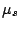, whose value is obtained from experiments or ab-initio calculations. The natural unit is the Bohr magnetonand the moment constitutes usually few magnetons. Instead of the vector, it is more convenient to work in reduced magnetic moment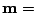.

From the magnetic point of view, the main energy contributions are the Zeeman energy, the magnetic dipole-dipole energy, the exchange energy and the magnetic anisotropy energy.

The anisotropy reflects the existence of preferred spatial directions in which the magnetization will align. There are different sources of anisotropy, but their contribution to the energy can always be written in similar functional dependence on. The principal source is the magnetocrystalline anisotropy that has its origin in the spin-orbit (LS) coupling and the ionic crystal field. Due to this fact, the magnetocrystalline anisotropy has the same symmetry as the lattice of the material. For uniaxial materials the anisotropy energy is an expansion of even powers of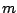that usually reduces to its first term:(2.2)

Its main effect is to align the magnetic moment in the direction of, which is known as easy axis. If the material has a cubic lattice the anisotropy is an expansion of terms with cubic symmetry and considering only its first term is equal to: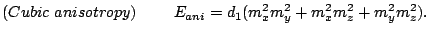(2.3)

The other sources of magnetic anisotropy are: surface anisotropy, magnetoelastic anisotropy and shape anisotropy.

The Zeeman energy is the energy of a magnetic dipole in a magnetic field: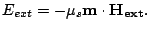(2.4)

The applied field tends to align the magnetization in its direction. Both the anisotropy and the Zeeman energies are local and are easy to calculate.

The exchange interaction is an electronic interaction whose origins are the Pauli exclusion principle and the Coulomb repulsion. The exchange interaction requires the integration of the electronic wave function. A more convenient evaluation of the exchange generally involves calculating the exchange explicitly using the Heisenberg form:(2.5)

withthe exchange integral. This definition of the Heisenberg exchange energy will be used throughout the thesis. This sum extends to all the moment pairs in the material, but due to the short range of the exchange interaction, the sum is usually restricted to nearest neighbors: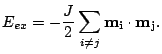(2.6)

A positive value of exchange parameter yields ferromagnetism and a negative value antiferromagnetism. The main effect of exchange is the alignment of all the magnetic moments in the case of ferromagnetism and it is usually the strongest interaction that dominates locally. However, the exchange interaction is isotropic and can not alone account for the ferromagnetism, thus needing the anisotropic energy term. We should note here that the Heisenberg form of exchange explicitly implies the direct exchange formation. Other forms of exchange interactions (indirect, RKKY, etc...) in same cases could be mapped into the Heisenberg form with some correction. Generally speaking, this is not true.

The interaction energy between two moments in the material is the classical dipole-dipole energy: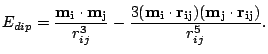(2.7)

The contribution of each of the individual terms is the least important of the energies. However, this does not mean that the dipolar interactions can be neglected because, being long-ranged, they are a sum over the whole magnetic material. The dipolar interaction is responsible for magnetic inhomogeneities, the foremost of them is the domain wall. The exchange controls the details of the microscopic magnetic structure, while the magnetostatic energy is responsible for the magnetization distribution over the whole system.

2008-04-04